# Prime Numbers.pptx

21 de Mar de 2023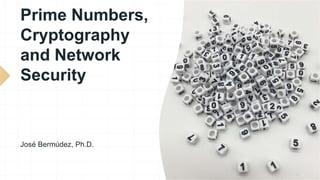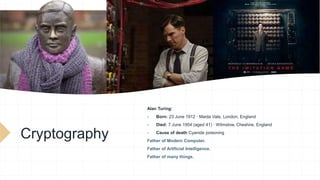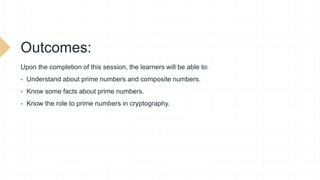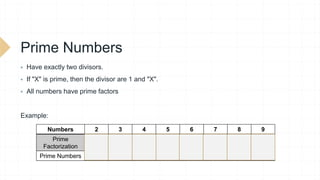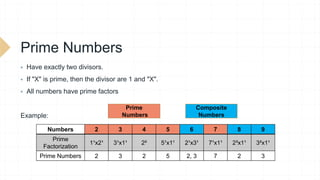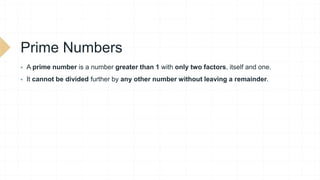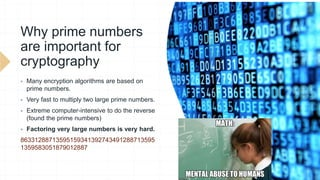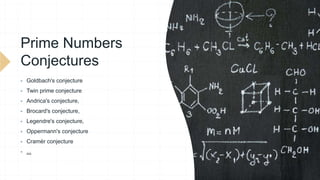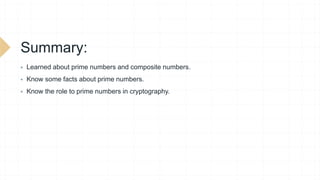1 de 10

### Prime Numbers.pptx

1. Prime Numbers, Cryptography and Network Security José Bermúdez, Ph.D.
2. Cryptography Alan Turing:  Born: 23 June 1912 · Maida Vale, London, England  Died: 7 June 1954 (aged 41) · Wilmslow, Cheshire, England  Cause of death Cyanide poisoning Father of Modern Computer. Father of Artificial Intelligence. Father of many things.
3. Outcomes: Upon the completion of this session, the learners will be able to:  Understand about prime numbers and composite numbers.  Know some facts about prime numbers.  Know the role to prime numbers in cryptography.
4. Prime Numbers  Have exactly two divisors.  If "X" is prime, then the divisor are 1 and "X".  All numbers have prime factors Example: Numbers 2 3 4 5 6 7 8 9 Prime Factorization 1¹x2¹ 3¹x1¹ 2² 5¹x1¹ 2¹x3¹ 7¹x1¹ 2³x1¹ 3²x1¹ Prime Numbers 2 3 2 5 2, 3 7 2 3
5. Prime Numbers  Have exactly two divisors.  If "X" is prime, then the divisor are 1 and "X".  All numbers have prime factors Example: Numbers 2 3 4 5 6 7 8 9 Prime Factorization 1¹x2¹ 3¹x1¹ 2² 5¹x1¹ 2¹x3¹ 7¹x1¹ 2³x1¹ 3²x1¹ Prime Numbers 2 3 2 5 2, 3 7 2 3 Prime Numbers Composite Numbers
6. Prime Numbers  A prime number is a number greater than 1 with only two factors, itself and one.  It cannot be divided further by any other number without leaving a remainder.
7. Why prime numbers are important for cryptography  Many encryption algorithms are based on prime numbers.  Very fast to multiply two large prime numbers.  Extreme computer-intensive to do the reverse (found the prime numbers)  Factoring very large numbers is very hard. 86331288713595159341392743491288713595 1359583051879012887
8. Prime Numbers Conjectures  Goldbach's conjecture  Twin prime conjecture  Andrica's conjecture,  Brocard's conjecture,  Legendre's conjecture,  Oppermann's conjecture  Cramér conjecture  ...
9. Summary:  Learned about prime numbers and composite numbers.  Know some facts about prime numbers.  Know the role to prime numbers in cryptography.
10. Prime Numbers, Cryptography and Network Security José Bermúdez, Ph.D.